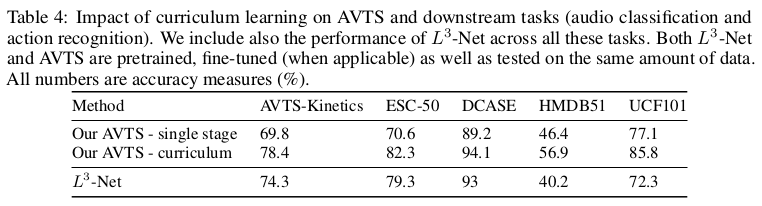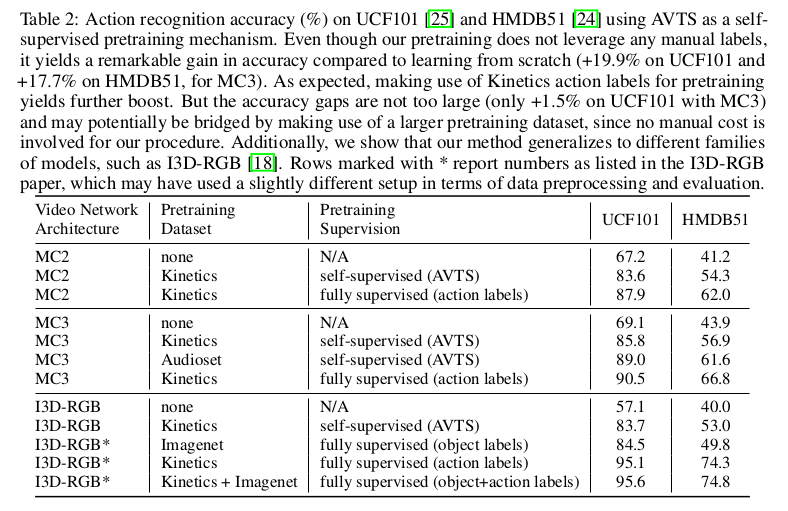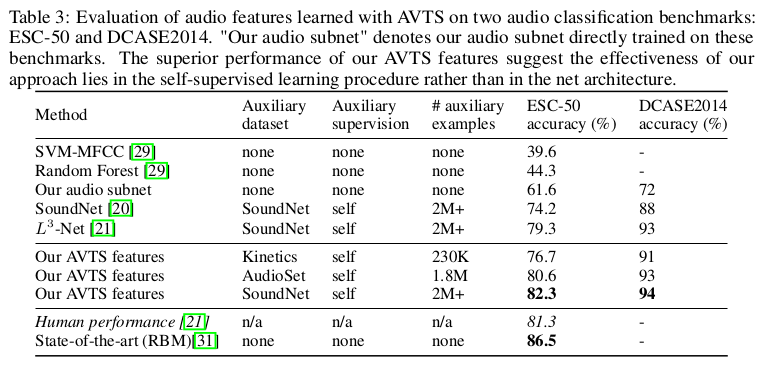# 论文引入

• 视觉听觉在语义和时序同步性判断
• 视听觉相关性判断，实现了自监督学习特征提取
• 预训练AVTS模型在视觉信息和听觉信息独立任务上取得了提高

# AVTS模型

AVTS模型是对视觉信息和听觉信息在语义和时序上同步性的判断，判断结果是二分类问题，要么同步要么不同步，我们先看一下模型框架：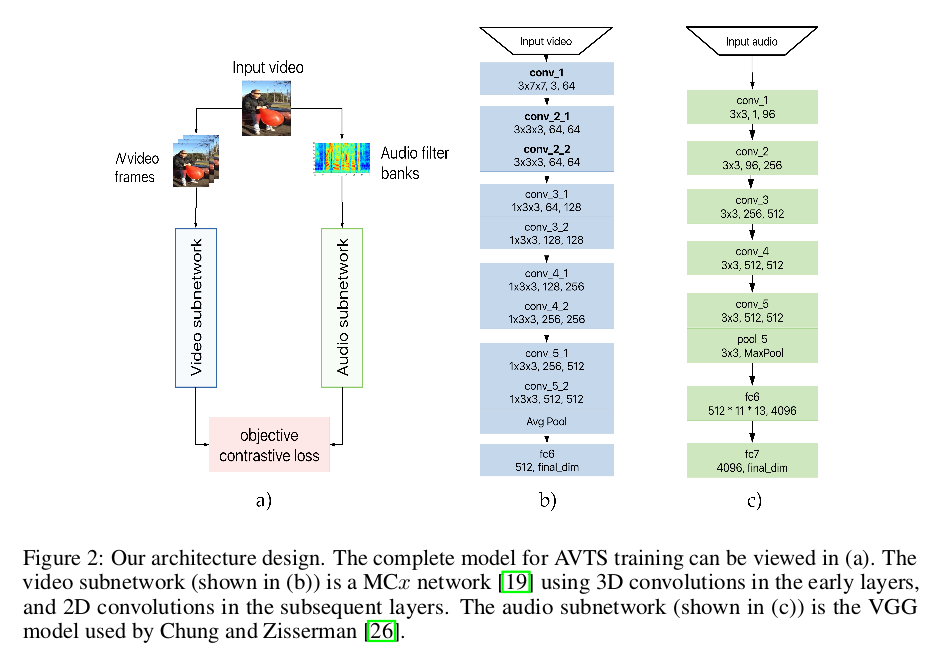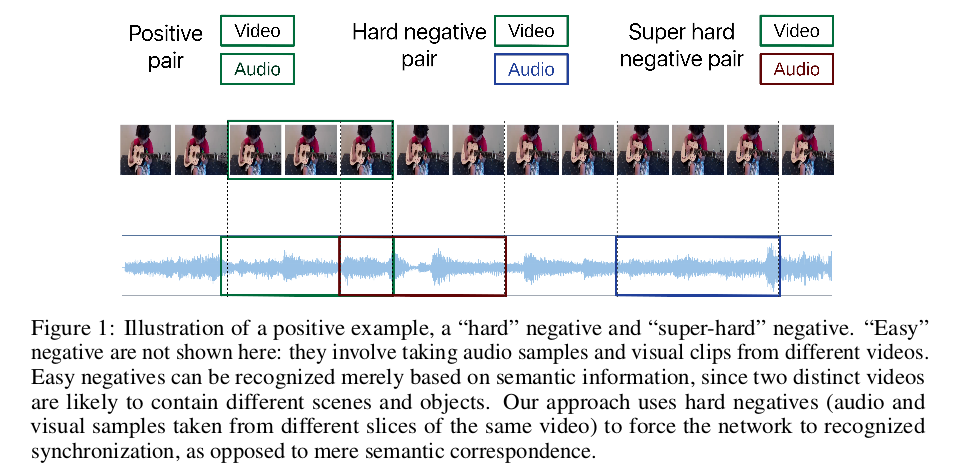$\begin{equation} E = \frac{1}{N} \sum_{n=1}^N (y^{(n)}) \Vert f_v(v^{(n)} - f_a(a^{(n)} \Vert_2^2) + (1-y^{(n)})max(\eta-\Vert f_v(v^{(n)} - f_a(a^{(n)} \Vert_2,0)^2 \end{equation}$

# 课程方式训练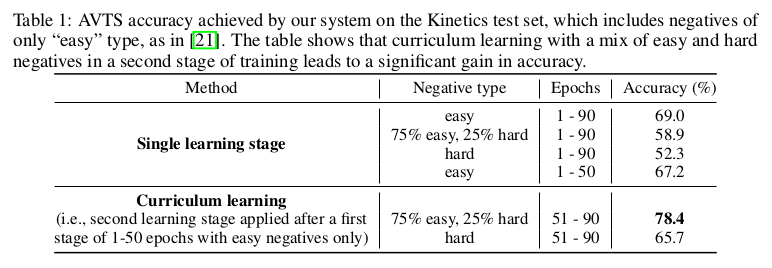# 实验